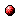DOC HOME SITE MAP MAN PAGES GNU INFO SEARCH

# (guile.info.gz) R5RS IndexInfo Catalog(guile.info.gz) Type Index(guile.info.gz) Top
```
R5RS Index
**********

[index]
```

```
* *                                     Arithmetic.          (line   6)
* +                                     Arithmetic.          (line   6)
* -                                     Arithmetic.          (line   6)
* /                                     Arithmetic.          (line   6)
* abs                                   Arithmetic.          (line   6)
* acos                                  Scientific.          (line  27)
* angle                                 Complex.             (line   6)
* append                                Append/Reverse.      (line  13)
* apply                                 Fly Evaluation.      (line  39)
* asin                                  Scientific.          (line  24)
* assoc                                 Retrieving Alist Entries.
(line   6)
* assq                                  Retrieving Alist Entries.
(line   6)
* assv                                  Retrieving Alist Entries.
(line   6)
* atan                                  Scientific.          (line  30)
* boolean?                              Booleans.            (line  65)
* call-with-current-continuation        Continuations.       (line  24)
* call-with-input-file                  File Ports.          (line  59)
* call-with-output-file                 File Ports.          (line  71)
* call-with-values                      Multiple Values.     (line  28)
* car                                   Pairs.               (line  62)
* cdr                                   Pairs.               (line  62)
* ceiling                               Arithmetic.          (line   6)
* char->integer                         Characters.          (line 112)
* char-alphabetic?                      Characters.          (line  81)
* char-ci<=?                            Characters.          (line  69)
* char-ci<?                             Characters.          (line  65)
* char-ci=?                             Characters.          (line  61)
* char-ci>=?                            Characters.          (line  77)
* char-ci>?                             Characters.          (line  73)
* char-downcase                         Characters.          (line 125)
* char-lower-case?                      Characters.          (line 101)
* char-numeric?                         Characters.          (line  86)
* char-upcase                           Characters.          (line 121)
* char-upper-case?                      Characters.          (line  96)
* char-whitespace?                      Characters.          (line  91)
* char<=?                               Characters.          (line  49)
* char<?                                Characters.          (line  46)
* char=?                                Characters.          (line  43)
* char>=?                               Characters.          (line  57)
* char>?                                Characters.          (line  53)
* char?                                 Characters.          (line  39)
* close-input-port                      Closing.             (line  14)
* close-output-port                     Closing.             (line  23)
* complex?                              Complex Numbers.     (line   6)
* cons                                  Pairs.               (line  45)
* cos                                   Scientific.          (line  18)
* current-input-port                    Default Ports.       (line   6)
* current-output-port                   Default Ports.       (line  12)
* display                               Writing.             (line  13)
* dynamic-wind                          Dynamic Wind.        (line   9)
* eq?                                   Equality.            (line  21)
* equal?                                Equality.            (line  33)
* eqv?                                  Equality.            (line  26)
* eval                                  Fly Evaluation.      (line   8)
* even?                                 Integer Operations.  (line   6)
* exact->inexact                        Exactness.           (line   6)
* exact?                                Exactness.           (line   6)
* exp                                   Scientific.          (line  33)
* expt                                  Scientific.          (line  12)
* floor                                 Arithmetic.          (line   6)
* for-each                              List Mapping.        (line  24)
* force                                 Delayed Evaluation.  (line  13)
* gcd                                   Integer Operations.  (line   6)
* imag-part                             Complex.             (line   6)
* inexact->exact                        Exactness.           (line   6)
* inexact?                              Exactness.           (line   6)
* input-port?                           Ports.               (line  20)
* integer->char                         Characters.          (line 117)
* integer?                              Integers.            (line   6)
* interaction-environment               Fly Evaluation.      (line  15)
* lcm                                   Integer Operations.  (line   6)
* length                                List Selection.      (line   9)
* list                                  List Constructors.   (line  11)
* list->string                          String Constructors. (line   9)
* list->vector                          Vector Creation.     (line  12)
* list-ref                              List Selection.      (line  18)
* list-tail                             List Selection.      (line  22)
* list?                                 List Predicates.     (line  11)
* log                                   Scientific.          (line  37)
* magnitude                             Complex.             (line   6)
* make-polar                            Complex.             (line   6)
* make-rectangular                      Complex.             (line   6)
* make-string                           String Constructors. (line  14)
* make-vector                           Vector Creation.     (line  40)
* map                                   List Mapping.        (line  13)
* max                                   Arithmetic.          (line   6)
* member                                List Searching.      (line  26)
* memq                                  List Searching.      (line  12)
* memv                                  List Searching.      (line  19)
* min                                   Arithmetic.          (line   6)
* modulo                                Integer Operations.  (line   6)
* negative?                             Comparison.          (line   6)
* newline                               Writing.             (line  21)
* not                                   Booleans.            (line  58)
* null?                                 List Predicates.     (line  20)
* number->string                        Conversion.          (line   6)
* number?                               Numerical Tower.     (line   6)
* odd?                                  Integer Operations.  (line   6)
* open-input-file                       File Ports.          (line  51)
* open-output-file                      File Ports.          (line  55)
* output-port?                          Ports.               (line  25)
* pair?                                 Pairs.               (line  51)
* positive?                             Comparison.          (line   6)
* procedure?                            Procedure Properties.
(line  20)
* quotient                              Integer Operations.  (line   6)
* rational?                             Reals and Rationals. (line   6)
* real-part                             Complex.             (line   6)
* real?                                 Reals and Rationals. (line   6)
* remainder                             Integer Operations.  (line   6)
* reverse                               Append/Reverse.      (line  34)
* round                                 Arithmetic.          (line   6)
* set-car!                              Pairs.               (line  75)
* set-cdr!                              Pairs.               (line  80)
* sin                                   Scientific.          (line  15)
* sqrt                                  Scientific.          (line   9)
* string                                String Constructors. (line   9)
* string->list                          List/String Conversion.
(line  11)
* string->number                        Conversion.          (line   6)
* string->symbol                        Symbol Primitives.   (line  45)
* string-append                         Appending Strings.   (line   9)
* string-ci<                            String Comparison.   (line  41)
* string-ci=?                           String Comparison.   (line  36)
* string-ci>=?                          String Comparison.   (line  54)
* string-ci>?                           String Comparison.   (line  50)
* string-copy                           String Selection.    (line  19)
* string-fill!                          String Modification. (line  15)
* string-length                         String Selection.    (line  10)
* string-ref                            String Selection.    (line  14)
* string-set!                           String Modification. (line  10)
* string<=?                             String Comparison.   (line  24)
* string<?                              String Comparison.   (line  20)
* string=?                              String Comparison.   (line  11)
* string>=?                             String Comparison.   (line  32)
* string>?                              String Comparison.   (line  28)
* string?                               String Predicates.   (line   9)
* substring                             String Selection.    (line  23)
* symbol->string                        Symbol Primitives.   (line  18)
* symbol?                               Symbol Primitives.   (line   9)
* tan                                   Scientific.          (line  21)
* truncate                              Arithmetic.          (line   6)
* values                                Multiple Values.     (line  20)
* vector                                Vector Creation.     (line  12)
* vector->list                          Vector Creation.     (line  28)
* vector-fill!                          Vector Accessors.    (line  42)
* vector-length                         Vector Accessors.    (line  10)
* vector-ref                            Vector Accessors.    (line  14)
* vector-set!                           Vector Accessors.    (line  34)
* vector?                               Vector Creation.     (line  49)
* with-input-from-file                  File Ports.          (line  83)
* with-output-to-file                   File Ports.          (line  94)
* write-char                            Writing.             (line  47)
* zero?                                 Comparison.          (line   6)

```Info Catalog(guile.info.gz) Type Index(guile.info.gz) Top
automatically generated byinfo2html